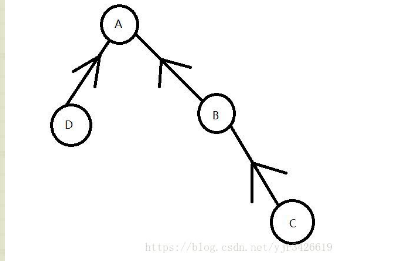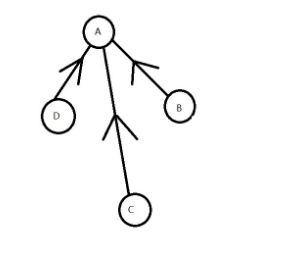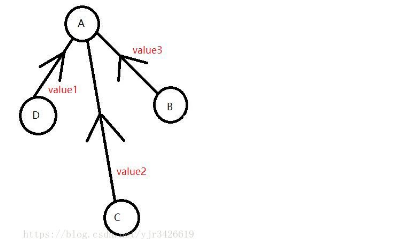# 学习笔记 带权并查集.

理解权值并查集我们首先需要知道并查集是什么，在这里简单说一下吧

普通并查集的主要作用是判断图是否连通，即两个节点之间是否存在某种联系（即同一个根节点），如下图所示就是一个很普通的并查集，只能看到边代表着方向额而没有记录其他信息

代码应该都会写，不上了但是有些时候我们需要判断更多的信息，比如把多个连通的图合并为一个图并且记录合并后节点的个数，我们就需要一个更为高级的结构，权值并查集

如何理解这个权值呢？这个权值的意思就是需要额外记录一些信息。

基于对并查集的理解，我们会发现一个比较有趣的事情，就是

看上面的图，我们对C进行find操作，让c连上A，不过在此之前，C会先找到B，然后才连上A，我们可不可以直接把C和A相连呢，这样极大程度上缩小了时间复杂度，得到的结果如下这种优化有一个学名，叫做并查集的路径压缩，将每一个节点直接与其find操作得到的节点连接。

很轻松的可以写出这样的代码

1 int find(int x)
2 {
3     if (x != f[x])
4     {
5         f[x] = find(f[x]);
6     }
7     return f[x];
8 }

和一般的并查集相比，这个不过是多了一个在find前面加了一个赋值操作，把所有要找的节点的根节点设成最后找到的那个点而已！！！但是就比之前的传统并查集优秀

基于路径压缩的原理，权值并查集就变成了一下的样子发现这个图，每一条边都记录了一条信息，具体记录什么信息需要依据题意而定，in general 都是一些边的相对关系。但是最主要的是，有权值就会有几个问题

1.由于我们优秀的路径压缩操作，所以我们记录的权值都是与根节点之间的权值，在我们find操作中，路径压缩也要更新这个权值

2.在并查集的合并操作中，我们也需要更新权值，因为根节点！！！路径压缩存储的是与根节点之间的权值！！！

所以呢，路径压缩的权值修改和并查集合并操作更新权值代码部分如下

（合并并查集）

1     x=read(),y=read();
2     int fx=find(x);
3     int fy=find(y);
4     if(fy!=fx)
5     {
6         f[fx]=fy;
7         sum[fx]=num[fy];
8         num[fy]+=num[fx];
9     }

（路径压缩权值修改）

1 int find(int x)
2 {
3     if(x==f[x])
4     return x;
5     int fa=find(f[x]);
6     sum[x]+=sum[f[x]];
7     return f[x]=fa;
8 }

是不是非常简单呢？？

23333上两道例题

#### 题目描述

约翰所在的乡村可以看做一个二维平面，其中有 NN

突然间，约翰的导航仪失灵了，牧场的坐标记录全部消失了。所幸的是，约翰找到了表示道路的数据，可以通过这些信息得知牧场间的相对位置。但贝西有急事，在约翰工作到一半的时候就要知道一些牧场间的曼哈顿距离。这时，如果约翰能从找回的道路信息之间推算出答案，就会告诉贝西。请你帮助约翰来回答贝西的问题吧。(x1,y1) (x_1, y_1)

第11

第22

第M+2M+2

第M+3M+3

#### 输出格式

• 对每个询问，输出牧场间的曼哈顿距离，如果当时恢复的数据还不足以得知答案，输出 1。以换行符分隔答案

#### 样例输入 1

　　7 6
1 6 13 E
6 3 9 E
3 5 7 S
4 1 3 N
2 4 20 W
4 7 2 S
3
1 6 1
1 4 3
2 6 6

#### 样例输出 1

　　13
-1
10

#### 样例解释1

　　在时刻1，约翰知道1到6的距离为13;
在时刻3，1到4的距离仍然不知道；
在时刻6，位置6向北3个距离，向西7个距离于位置2，所以距离为10.

AC代码如下1 #include <bits/stdc++.h>
2 #define ll long long
3 #define res register
4 #define MAXN 100050
5 using namespace std;
6 int f[MAXN],nx[MAXN],ny[MAXN];
7 int n,m,q;
8 char s;
9 int find(int x)
10 {
11     int y=f[x];
12     if(x!=f[x])
13     f[x]=find(f[x]);
14     nx[x]+=nx[y];
15     ny[x]+=ny[y];
16     return f[x];
17 }
18 struct Node
19 {
20     int x,y,c,p;
21     int ans;
22     inline friend bool operator <(Node a,Node b)
23     {
24         return a.c<b.c;
25     }
26 }t,tt;
27 bool cmp(Node a,Node b)
28 {
29     return a.p<b.p;
30 }
32 {
33     int s=0,w=1;char ch=getchar();
34     while(ch<'0'||ch>'9'){if(ch=='-')w=-1;ch=getchar();}
35     while(ch>='0'&&ch<='9'){s=(s<<1)+(s<<3)+ch-48;ch=getchar();}
36     return s*w;
37 }
38 int main()
39 {
40     freopen("navigate.in","r",stdin);
41     freopen("navigate.out","w",stdout);
43     for(res int i=1;i<=n;i++)
44     f[i]=i,nx[i]=ny[i]=0;
45     for(res int i=1;i<=m;i++)
46     {
47         int x,y,c;
49         scanf("%s",s);
50         t[i].x=x;
51         t[i].y=y;
52         t[i].c=c;
53         if(s=='E')
54         t[i].p=0;
55         else if(s=='W')
56         t[i].p=1;
57         else if(s=='N')
58         t[i].p=2;
59         else
60         t[i].p=3;
61     }
63     for(res int i=1;i<=q;i++)
64     {
65         int x,y,c;
67         tt[i].x=x;
68         tt[i].y=y;
69         tt[i].c=c;
70         tt[i].p=i;
71     }
72     sort(tt+1,tt+1+q);
73     int now=0;
74     for(int i=1;i<=q;i++)
75     {
76         while(now<tt[i].c)
77         {
78             now++;
79             int x=t[now].x,y=t[now].y,c=t[now].c;
80             int ff=find(y);
81             nx[ff]=-nx[y];
82             ny[ff]=-ny[y];
83             f[ff]=y;
84             nx[y]=ny[y]=0;
85             f[y]=x;
86             if(t[now].p==0)
87             nx[y]=c;
88             else if(t[now].p==1)
89             nx[y]=-c;
90             else if(t[now].p==2)
91             ny[y]=c;
92             else
93             ny[y]=-c;
94         }
95         int x=tt[i].x,y=tt[i].y;
96         if(find(x)!=find(y)) tt[i].ans=-1;
97         else
98         {
99             tt[i].ans=abs(nx[x]-nx[y])+abs(ny[x]-ny[y]);
100         }
101     }
102     sort(tt+1,tt+1+q,cmp);
103     for(int i=1;i<=q;i++)
104     printf("%d\n",tt[i].ans);
105     return 0;
106 }
View Code

posted @ 2019-08-22 14:36  Lbmttw_lx  阅读(288)  评论(1编辑  收藏  举报